# nLab cohomotopy

Contents

cohomology

## Theorems

#### Spheres

n-sphere

low dimensional n-spheres

# Contents

## Idea

### General

Cohomotopy cohomology theory $\pi^\bullet$ is the (non-abelian) generalized cohomology theory whose cocycle spaces are spaces of maps into an n-sphere, hence whose cohomology classes are homotopy classes of maps into an n-sphere:

$\pi^n(X) \;\coloneqq\; Maps\big( X, S^n\big)/_{\sim_{homotopy}}$

So, dually to how homotopy groups

$\pi_n(X)\coloneqq Maps\big( S^n, X\big)/_{\sim_{homotopy}}$

are groups of homotopy classes of maps out of spheres, Cohomotopy sets are sets of homotopy classes of maps into spheres, whence the dual name.

If instead one considers only the stable aspect of Cohomotopy sets, by mapping into the stabilization of the spheres, hence into (some suspension of) the sphere spectrum, then one speaks of stable Cohomotopy, written

$\mathbb{S}^n(X) \;\coloneqq\; Maps \big( \Sigma^\infty X , \Sigma^{\infty} S^n \big)/_{\sim_{homotopy}} \,.$

In other words, the generalized (Eilenberg-Steenrod) cohomology theory which is represented by the sphere spectrum is stable Cohomotopy.

###### Remark

(terminology)

Therefore “Cohomotopy theory” is really shorthand for “Cohomotopy cohomology theory” and as such is dual to homotopy homology theory, which in the stable case is known as stable homotopy homology theory.

In particular, cohomotopy theory is a concrete particular and not dual to the abstract general of homotopy theory; and is hence also not on par with the abstract general of cohomology theory. Rather, Cohomotopy theory is one instance of a cohomology theory, and as such is a sibling of ordinary cohomology theory (HR-theory)), K-theory, etc.

To emphasize this, one might, in the stable case, say $\mathbb{S}$-theory instead of “stable Cohomotopy theory”; where $\mathbb{S}$ denotes the sphere spectrum. In the unstable case there is no widely adopted notation, but one might consider saying “$\mathbf{\pi}$-theory” (with $\pi$ the established symbol for (co)homotopy groups) or $S$-theory (with “$S$” for n-spheres $S^n$) for unstable Cohomotopy theory.

In any case, to highlight that Cohomotopy theory is a concrete particular and not an abstract general, it makes good sense to capitalize the term and speak of Cohomotopy cohomology theory or just Cohomotopy theory, for short.

The following table indicates the pattern:

generalized cohomology theorygeneralized homology theoryclassifying spacerepresenting spectrum
Cohomotopy theory
(non-abelian)
n-sphere
$S^n$
stable Cohomotopy theory
(abelian)
stable homotopy homology theorysphere spectrum
$\mathbb{S}$
$\,$
ordinary cohomology:
HR-cohomology theory
ordinary homology: HR-homology theoryEilenberg-MacLane space $K(R,n)$Eilenberg-MacLane spectrum
$H R$
K-cohomology theoryK-homology theorystable unitary group
BU
K-theory spectrum
KU, …

As for any generalized cohomology theory there are immediate variants to plain Cohomotopy theory, as shown in the following table:

flavours of
Cohomotopy
cohomology theory
cohomology
(full or rational)
equivariant cohomology
(full or rational)
non-abelian cohomologyCohomotopy
(full or rational)
equivariant Cohomotopy
twisted cohomology
(full or rational)
twisted Cohomotopytwisted equivariant Cohomotopy
stable cohomology
(full or rational)
stable Cohomotopyequivariant stable Cohomotopy

### As the absolute cohomology theory

In some sense Cohomotopy is the most fundamental of all generalized cohomology theories (“The Music of the Spheres”).

Concretely, stable Cohomotopy cohomology theory is the initial object among multiplicative cohomology theories, in that the sphere spectrum is the initial object in (E-infinity) ring spectra. This means that for any other multiplicative cohomology theory $E$ there is an essentially unique multiplicative natural transformation

(1)$\mathbb{S}^\bullet(X) \overset{\beta(X)}{\longrightarrow} E^\bullet(X)$

from Cohomotopy cohomology groups to $E$-cohomology groups – the Boardman homomorphism.

Specifically for $E = K \mathbb{F}$ the algebraic K-theory of a field $\mathbb{F}$ (such as a prime field $\mathbb{F}_p$) there is such a comparison morphism; and another way how stable Cohomotopy is the most fundamental of all K-theories is that it is equivalently the algebraic K-theory over the “absolute base”, namely over the “field with one element$\mathbb{F}_1$ (see there for more):

$\mathbb{S}^\bullet(X) \;\simeq\; K\mathbb{F}_1 ^ \bullet(X) \,.$

For example, for $E =$ KU and in the case of $G$-equivariant cohomology theory (equivariant Cohomotopy theory and equivariant K-theory) the Boardman homomorphism (1) gives the comparison map

$\mathbb{S}^0_G(\ast) \simeq R_{\mathbb{F}_1}(G) \simeq A(G) \overset{\mathbb{C}[-]}{\longrightarrow} R_{\mathbb{C}}(G) \simeq KU^0_G(\ast)$

from the Burnside ring to the representation ring of the finite group $G$, by forming permutation representations; where we may think of the Burnside ring as being the representation ring over the “field with one element” (see e.g. Chu-Lorscheid-Santhanam 10), as indicated above.

So far, this applies to stable Cohomotopy theory, which historically has received almost all the attention. But, while stabilization makes the immensely rich nature of homotopy theory a tad more tractable, it is only an approximation (just the first Goodwillie derivative!) of full unstable/non-abelian cohomology. Hence the one concept more fundamental than stable Cohomotopy theory is actual Cohomotopy theory.

For example, the classification of Yang-Mills instantons on $\mathbb{R}^4$ is typically regarded in the non-abelian cohomology theory represented by the classifying space $B SU(N)$ of the special unitary group (for $N \geq 2$, starting with SU(2))

$(B SU(N))^0 \Big( \big( \mathbb{R}^{4} \big)^{cpt} \Big) \;\coloneqq\; \Big\{ \big( \mathbb{R}^{4} \big)^{cpt} \to B SU(N) \Big\}/_{\sim_{homotopy}} \;\simeq\; \underset{ \mathclap{ \color{blue} {\text{instanton} \atop \text{number}} } }{ \mathbb{Z} } \,.$

But since the one-point compactification of 4d Euclidean space is the 4-sphere $\big( \mathbb{R}^4\big)^{cpt} \simeq S^4$, this classification factors through one in unstable Cohomotopy theory, via the “unstable Boardman homomorphism” $S^4 \longrightarrow B SU(N)$ representing the generator of the 4th homotopy group of $B SU(N)$ (see there)

$\pi^0 \Big( \big( \mathbb{R}^{4} \big)^{cpt}7 \Big) \;\coloneqq\; \Big\{ \big( \mathbb{R}^{4} \big)^{cpt} \to S^4 \Big\}/_{\sim_{homotopy}} \;\simeq\; \underset{ \mathclap{ \color{blue} {\text{instanton} \atop \text{number}} } }{ \mathbb{Z} } \,.$

(see SS 19, p. 9-10) This is the tip of an iceberg. Which needs to be discussed elsewhere.

## Properties

### Hopf degree theorem

###### Proposition

(Hopf degree theorem)

Let $n \in \mathbb{N}$ be a natural number and $X \in Mfd$ be a connected orientable closed manifold of dimension $n$. Then the $n$th cohomotopy classes $\left[X \overset{c}{\to} S^n\right] \in \pi^n(X)$ of $X$ are in bijection to the degree $deg(c) \in \mathbb{Z}$ of the representing functions, hence the canonical function

$\pi^n(X) \underoverset{\simeq}{S^n \to K(\mathbb{Z},n)}{\longrightarrow} H^n(X,\mathbb{Z}) \;\simeq\; \mathbb{Z}$

from $n$th cohomotopy to $n$th integral cohomology is a bijection.

(e.g. Kosinski 93, IX (5.8))

### Relation to Freudenthal suspension theorem

relation to the Freudenthal suspension theorem (Spanier 49, section 9)

### Smooth representatives

For $X$ a compact smooth manifold, there is a smooth function $X \to S^n$ representing every cohomotopy class (with respect to the standard smooth structure on the sphere manifold).

### Reduced Cohomotopy

For some purposes (such as in stating Pontryagin's theorem below) it is more natural to use reduced Cohomotopy:

For $(X,x)$ a pointed homotopy type/cofibrant pointed topological space, its reduced $n$-Cohomotopy is the set of connected components in the mapping space of pointed functions to the n-sphere (for any choice of basepoint on the latter, then naturally thought of as $\infty \in (\mathbb{R}^n)^{cpt} \simeq S^n$):

(2)${\widetilde \pi}{}^n (X,x) \;\coloneqq\; \pi_0 Maps^{\ast/} \big( (X,x), (S,\infty) \big) \,.$

However, the difference between reduced and unreduced Cohomotopy is small:

###### Proposition

For $n \geq 1$ a positive natural number and $(X,x)$ a pointed topological space/pointed homotopy type, the canonical morphism from reduced (2) to unreduced Cohomotopy of $X$ in degree $n$ is an isomorphism:

${\widetilde \pi}^{n \geq 1}(X,x) \overset{\;\;\simeq\;\;}{\longrightarrow} {\pi}^{n \geq 1}(X) \,.$

(e.g. Hatcher, 4A.2, 4A.3)
###### Proof

Observe that

$Maps^{\ast/}\big((X,x),(S^n,\infty) \big) \overset{fib(ev_x)}{\longrightarrow} Maps\big(X,S^n \big) \overset{ev_x}{\longrightarrow} S^n$

(where $ev_x$ is the evaluation map at $x \in X$) is a homotopy fiber sequence as soon as $X$ is represented by a cell complex (e.g. CW-complex). This follows either from the pullback-power axiom in the classical model structure on topological spaces, or, more abstractly from the characterization of derived hom-spaces in coslice (infinity,1)-categories here.

Therefore we have the corresponding long exact sequence of homotopy groups, which starts out as

By exactness, this yields the claim for $n \geq 2$; while for $n = 1$ it gives the desired isomorphism only up to a possibly non-trivial quotient by an action of $\mathbb{Z} \simeq \pi_1(S^1)$:

${\widetilde \pi}{}^1(X,x)/\pi_1(S^1) \simeq \pi^1(X) \,.$

Hence it is now sufficient to see that the $\pi_1(S^1)$-action on reduced 1-Cohomotopy is always trivial:

Now, the action of $n \in \pi_1(S^1)$ takes $\big[ (X,x) \overset{ \;c\; }{\longrightarrow} (S^1,\ast) \big]$ to any $\big[ (X,x) \overset{ \;c'\; }{\longrightarrow} (S^1,\ast) \big]$ such that $[X \overset{c}{\longrightarrow} S^1] \overset{\eta}{\Rightarrow} [X \overset{c'}{\longrightarrow} S^1]$ in $\pi^1(X)$, with $[\eta(x,-)] = n \in \pi_1(S^1)$. But using the group-structure on $S^1 = \mathbb{R}/\mathbb{Z}$ we have that one such $\eta$ is given by $\eta(x,t) \coloneqq c(x) + n t \,mod\, \mathbb{Z}$, which yields $c'(x) = c(x) + n \,mod\, \mathbb{Z}$ and hence $c' = c$.

### Pontryagin isomorphism between Cohomotopy and framed Cobordism

Pontryagin's theorem (Pontryagin 38, Pontryagin 55) is an identification of cobordism classes of normally framed submanifolds of codimension $n$ in a closed manifold $X^d$ with the $n$-Cohomotopy of that manifold – the homotopy classes of maps into the n-sphere, which is exhibited by the Cohomotopy charge map, often known as the Pontryagin-Thom collapse construction:

$Cob^n_Fr(X) \overset{\simeq}{\longrightarrow} \pi^n(X) \,.$

The analogous statement identifying cobordism classes of normally oriented submanifolds with homotopy classes of maps into the universal special orthogonal Thom space $M SO(n)$ is Thom's theorem (Thom 54). Both statements, as well as their joint generalization to other tangential structures and notably their stabilization to Whitehead-generalized Cobordism cohomology theory, have come to be widely known as the Pontryagin-Thom collapse construction, or similar, and form the basis of modern cobordism theory and its application in stable homotopy theory.

For $X$ a closed smooth manifold of dimension $D$, the assignment of Cohomotopy charge (the Pontryagin-Thom construction, in this unstable and framed version due to Pontrjagin 55, see review in Kosinski 93, IX.5, Milnor 97) identifies the set

$SubMfd_{/bord}^{d}(X)$

of cobordism classes of closed and normally framed submanifolds $\Sigma \overset{\iota}{\hookrightarrow} X$ of dimension $d$ inside $X$ with the cohomotopy $\pi^{D-d}(X)$ of $X$ in degree $D- d$

$SubMfd_{/bord}^{d}(X) \underoverset{\simeq}{\;\;\;PT\;\;\;}{\longrightarrow} \pi^{D-d}(X) \,.$

In particular, by this bijection the canonical group structure on cobordism groups in sufficiently high codimension (essentially given by disjoint union of submanifolds) this way induces a group structure on the cohomotopy sets in sufficiently high degree.

$\,$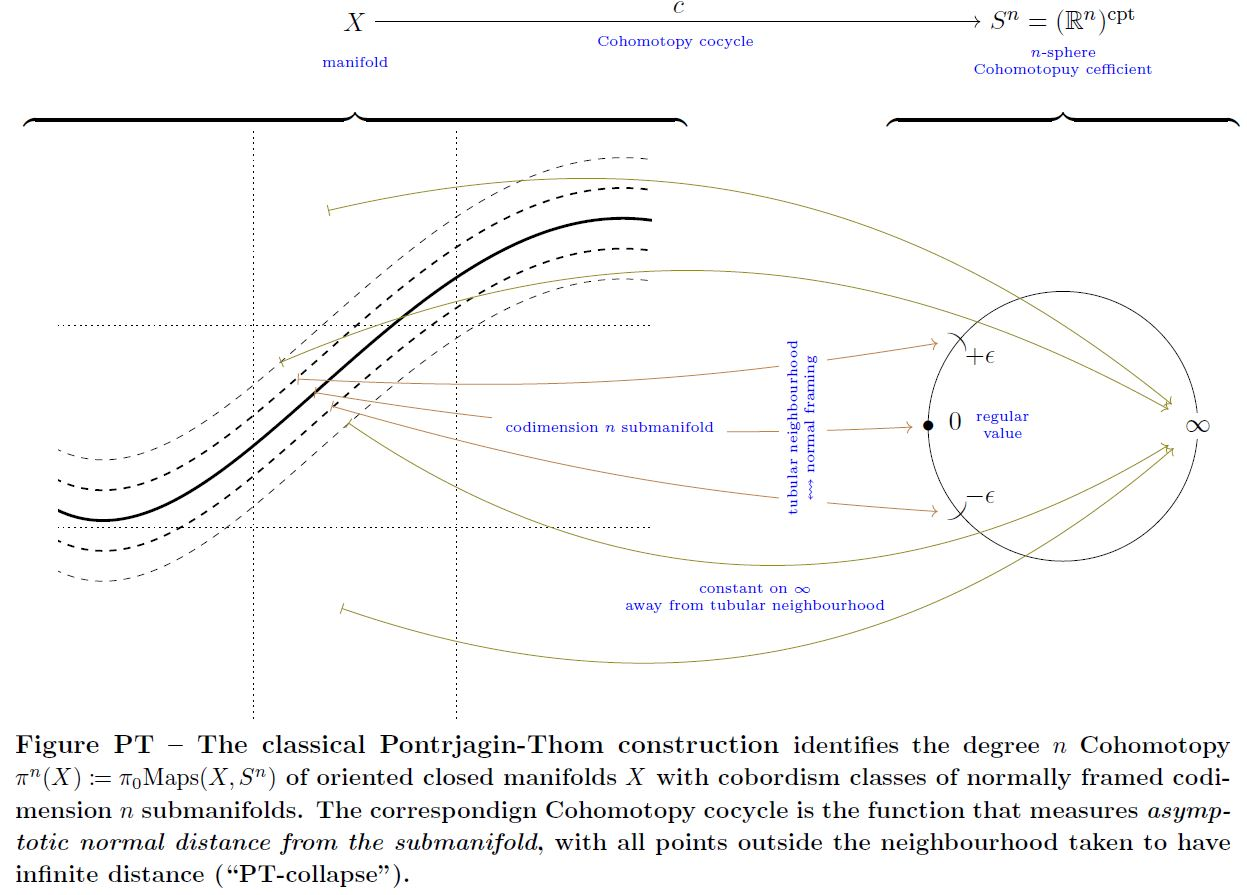graphics grabbed form Sati-Schreiber 19

Here the normal framing of the submanifolds plays the role of the charge in Cohomotopy which they carry:

$\,$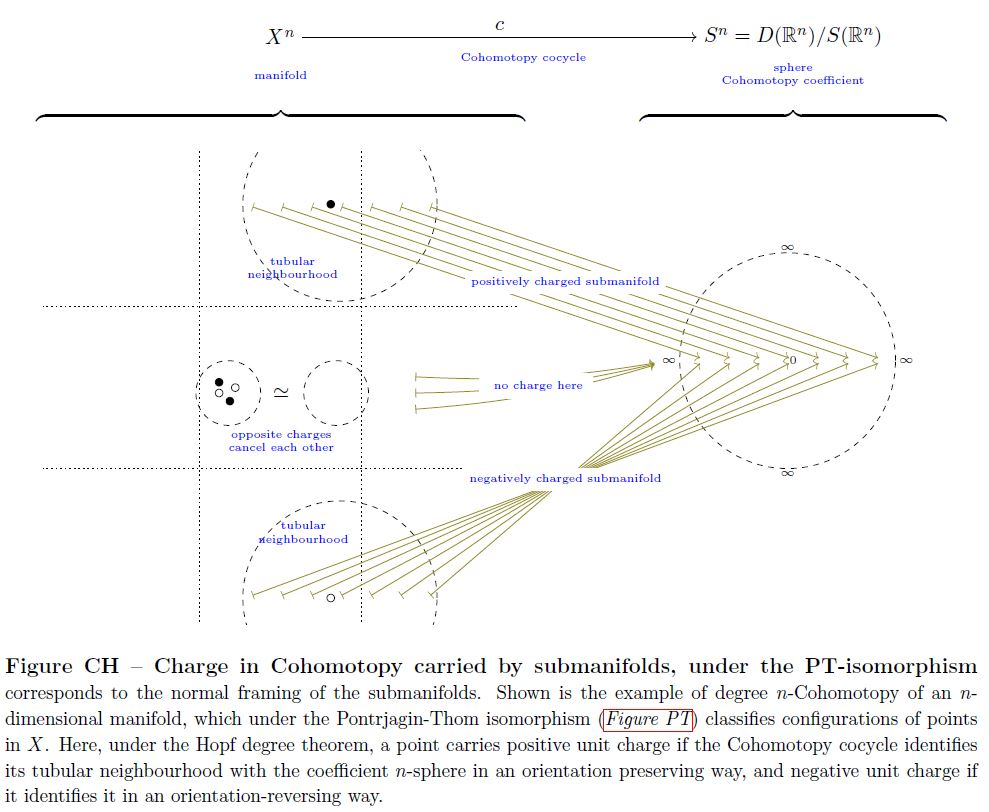graphics grabbed form Sati-Schreiber 19

For example:

$\,$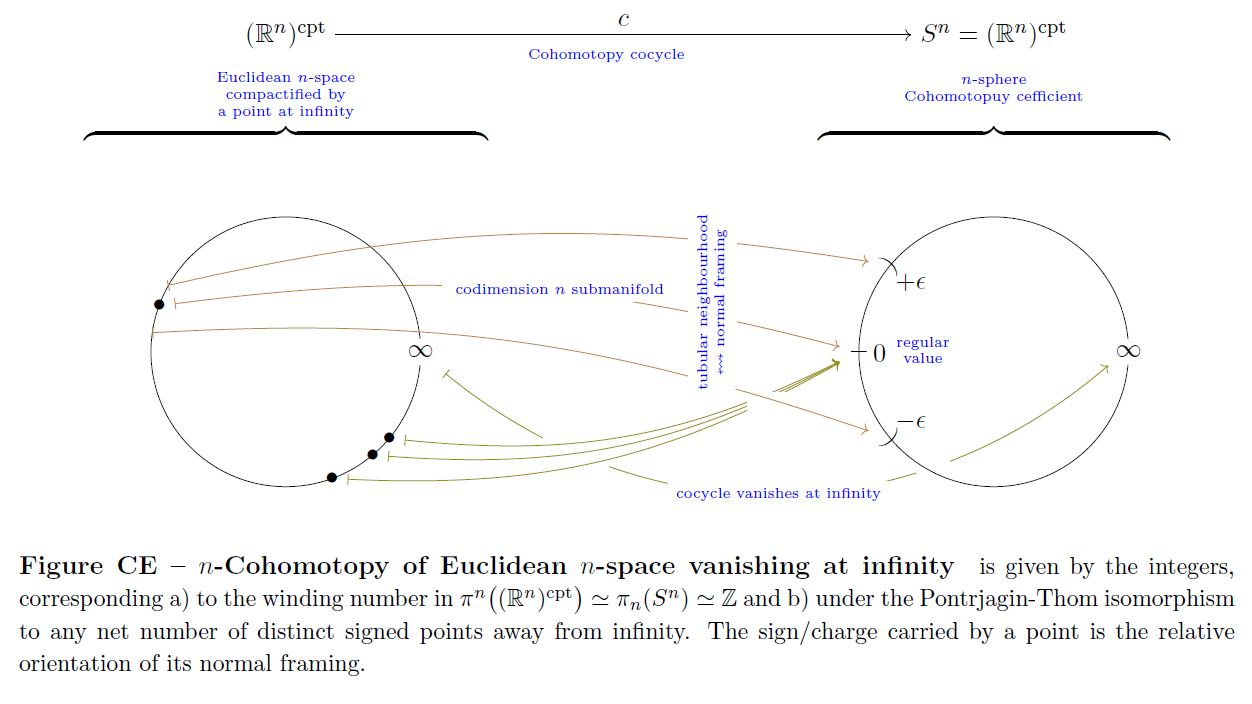graphics grabbed form Sati-Schreiber 19

This construction generalizes to equivariant cohomotopy, see there.

With the equivariant Hopf degree theorem the above example has the following $\mathbb{Z}_2$-equivariant version (see there):

$\,$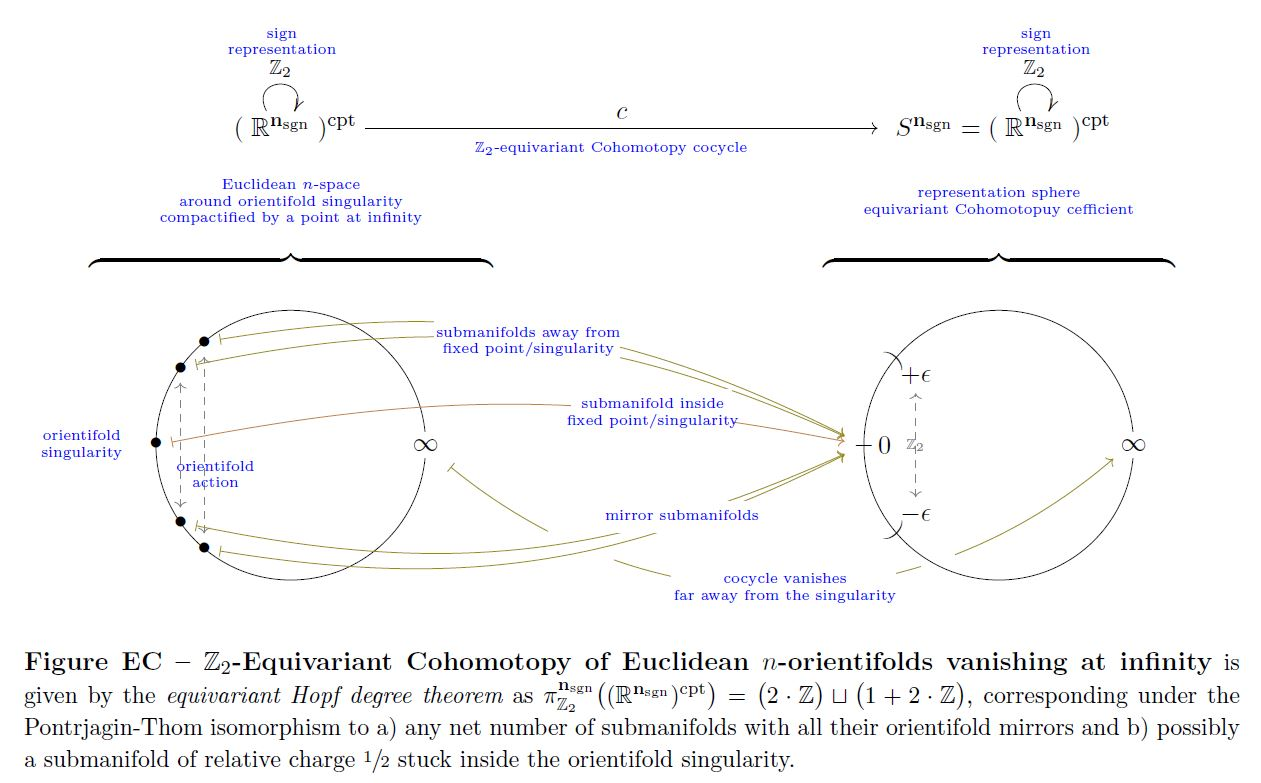graphics grabbed form Sati-Schreiber 19

Further by the equivariant Hopf degree theorem (see there), this example generalizes to equivariant cohomotopy of toroidal orientifolds:

$\,$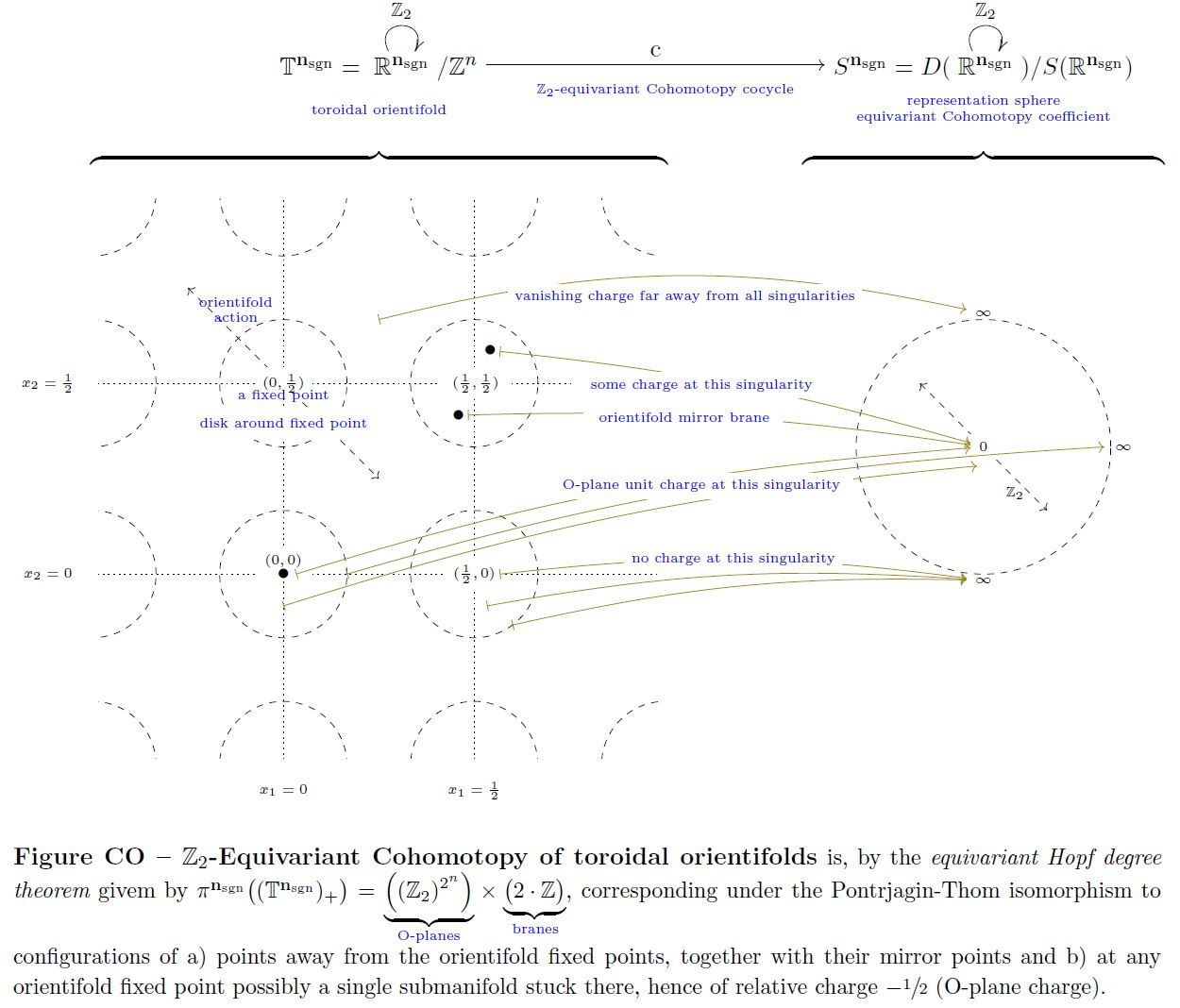graphics grabbed form Sati-Schreiber 19

### Composition/product in Cohomotopy

Under the Pontryagin-Thom isomorphism (above) the product in Cohomotopy, i.e. the composition operation

$\array{ \pi^{n_1} \big( S^{n_2 + n_1} \big) \times \pi^{n_2 + n_1}(X) &\overset{}{\longrightarrow}& \pi^{n_1}(X) \\ (c_1, c_2) &\mapsto& c_1 \circ c_2 } \,,$

corresponds to forming product manifolds of submanifolds, in that (see also Kosinski 93, Section IX 6.1):

$\mathrm{PT}^{-1}(c_1 \circ c_2) \;\simeq\; \Big[ \mathrm{PT}^{-1}(c_2) \times \mathrm{PT}^{-1}(c_1) \;\subset\; \mathrm{PT}^{-1}(c_2) \times \mathbb{R}^{n_2 + n_1} \;\subset\; X \Big] \,.$

This is exhibited by the following pasting diagram of pullbacks/fiber products (repeatedly using the pasting law):

Here the vertical inclusions are the defining ones of the submanifolds or of their normal bundles, identified with some choice of tubular neighbourhoods.

### Cohomotopy charge map and Relation to configuration spaces

The Cohomotopy charge map is the function that assigns to a configuration of points their total charge as measured in Cohomotopy-cohomology theory.

This is alternatively known as the “electric field map” (Salvatore 01 following Segal 73, Section 1, see also Knudsen 18, p. 49) or the “scanning map” (Kallel 98).

For $D \in \mathbb{N}$ the Cohomotopy charge map is the continuous function

(3)$Conf\big( \mathbb{R}^D \big) \overset{cc}{\longrightarrow} \mathbf{\pi}^D \Big( \big( \mathbb{R}^D \big)^{cpt} \Big) = Maps^{\ast/\!}\Big( \big(\mathbb{R}^D\big)^{cpt} , S^D\big) = \Omega^{D} S^D$

from the configuration space of points in the Euclidean space $\mathbb{R}^D$ to the $D$-Cohomotopy cocycle space vanishing at infinity on the Euclidean space(which is equivalently the space of pointed maps from the one-point compactification $S^D \simeq \big( \mathbb{R}^D \big)$ to itself, and hence equivalently the $D$-fold iterated based loop space of the D-sphere), which sends a configuration of points in $\mathbb{R}^D$, each regarded as carrying unit charge to their total charge as measured in Cohomotopy-cohomology theory (Segal 73, Section 3).

This has evident generalizations to other manifolds than just Euclidean spaces, to spaces of labeleed configurations and to equivariant Cohomotopy. The following graphics illustrates the Cohomotopy charge map on G-space tori for $G = \mathbb{Z}_2$ with values in $\mathbb{Z}_2$-equivariant Cohomotopy: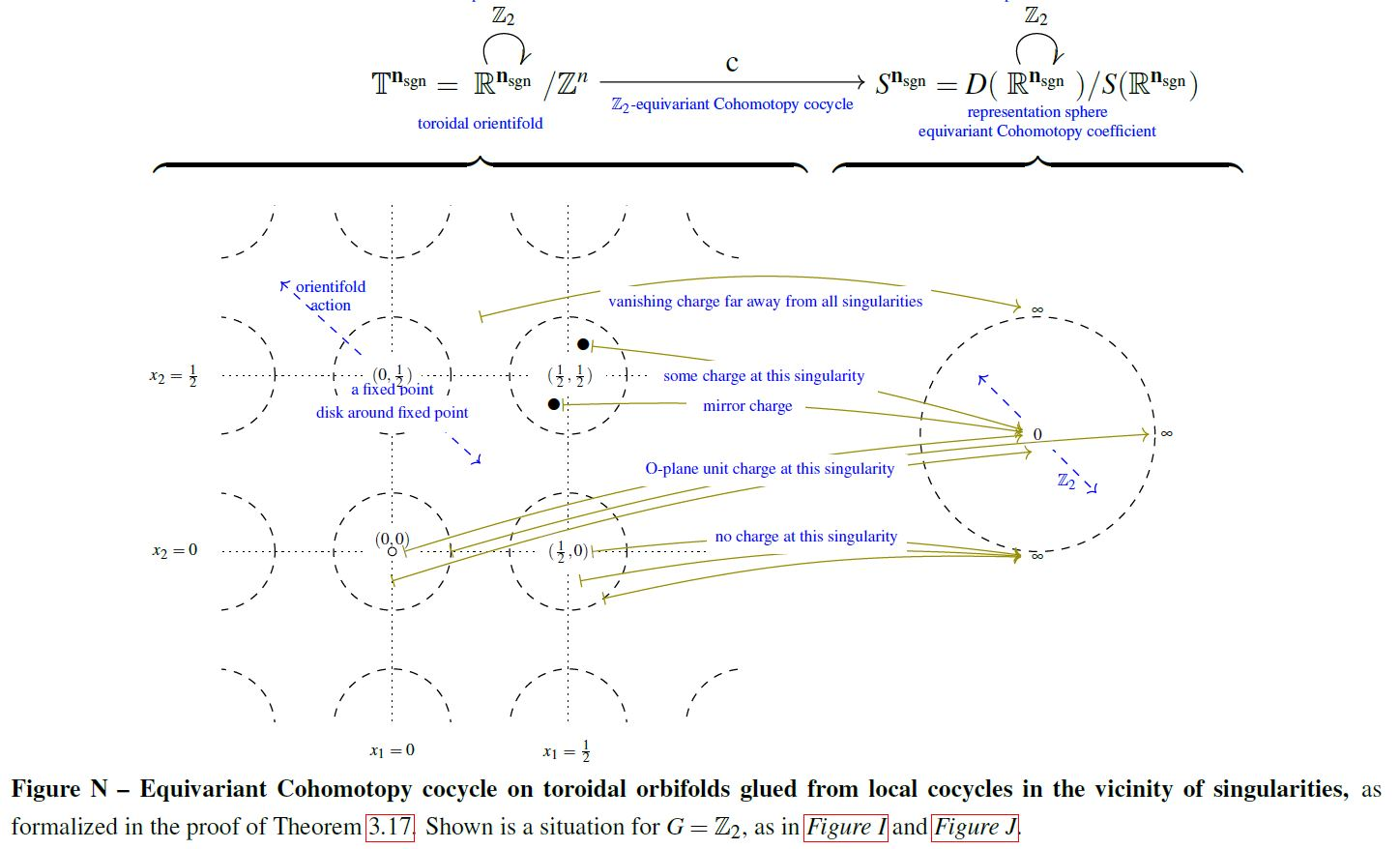graphics grabbed from SS 19

In some situations the Cohomotopy charge map is a weak homotopy equivalence and hence exhibits, for all purposes of homotopy theory, the Cohomotopy cocycle space of Cohomotopy charges as an equivalent reflection of the configuration space of points.

###### Proposition

(group completion on configuration space of points is iterated based loop space)

$Conf \big( \mathbb{R}^D \big) \overset{ cc }{\longrightarrow} \Omega^D S^D$

from the full unordered and unlabeled configuration space (here) of Euclidean space $\mathbb{R}^D$ to the $D$-fold iterated based loop space of the D-sphere, exhibits the group completion (here) of the configuration space monoid

$\Omega B_{{}_{\sqcup}\!} Conf \big( \mathbb{R}^D \big) \overset{ \simeq }{\longrightarrow} \Omega^D S^D$
###### Proposition

(Cohomotopy charge map is weak homotopy equivalence on sphere-labeled configuration space of points)

For $D, k \in \mathbb{N}$ with $k \geq 1$, the Cohomotopy charge map (3)

$Conf \big( \mathbb{R}^D, S^k \big) \underoverset{\simeq}{cc}{\longrightarrow} \Omega^D S^{D + k}$

is a weak homotopy equivalence from the configuration space (here) of unordered points with labels in $S^k$ and vanishing at the base point of the label space to the $D$-fold iterated loop space of the D+k-sphere.

The May-Segal theorem generalizes from Euclidean space to closed smooth manifolds if at the same time one passes from plain Cohomotopy to twisted Cohomotopy, twisted, via the J-homomorphism, by the tangent bundle:

###### Proposition

Let

1. $X^n$ be a smooth closed manifold of dimension $n$;

2. $1 \leq k \in \mathbb{N}$ a positive natural number.

Then the Cohomotopy charge map constitutes a weak homotopy equivalence

$\underset{ \color{blue} { \phantom{a} \atop \text{ J-twisted Cohomotopy space}} }{ Maps_{{}_{/B O(n)}} \Big( X^n \;,\; S^{ \mathbf{n}_{def} + \mathbf{k}_{\mathrm{triv}} } \!\sslash\! O(n) \Big) } \underoverset {\simeq} { \color{blue} \text{Cohomotopy charge map} } {\longleftarrow} \underset{ \mathclap{ \color{blue} { \phantom{a} \atop { \text{configuration space} \atop \text{of points} } } } }{ Conf \big( X^n, S^k \big) }$

between

1. the J-twisted (n+k)-Cohomotopy space of $X^n$, hence the space of sections of the $(n + k)$-spherical fibration over $X$ which is associated via the tangent bundle by the O(n)-action on $S^{n+k} = S(\mathbb{R}^{n} \times \mathbb{R}^{k+1})$

2. the configuration space of points on $X^n$ with labels in $S^k$.

(Bödigheimer 87, Prop. 2, following McDuff 75)

###### Remark

In the special case that the closed manifold $X^n$ in Prop. is parallelizable, hence that its tangent bundle is trivializable, the statement of Prop. reduces to this:

Let

1. $X^n$ be a parallelizable closed manifold of dimension $n$;

2. $1 \leq k \in \mathbb{N}$ a positive natural number.

Then the Cohomotopy charge map constitutes a weak homotopy equivalence

$\underset{ \color{blue} { \phantom{a} \atop \text{ Cohomotopy space}} }{ Maps \Big( X^n \;,\; S^{ n + k } \Big) } \underoverset {\simeq} { \color{blue} \text{Cohomotopy charge map} } {\longleftarrow} \underset{ \mathclap{ \color{blue} { \phantom{a} \atop { \text{configuration space} \atop \text{of points} } } } }{ Conf \big( X^n, S^k \big) }$

between

1. $(n+k)$-Cohomotopy space of $X^n$, hence the space of maps from $X$ to the (n+k)-sphere

2. the configuration space of points on $X^n$ with labels in $S^k$.

### Complex-rational Cohomotopy and moduli space of Yang-Mills monopoles

The assignment of scattering amplitudes of monopoles in SU(2)-Yang-Mills theory is a diffeomorphism

$\mathcal{M}_k \overset{ S }{\longrightarrow} R_k$

identifying the moduli space of monopoles of number $k$ with the space of complex-rational functions form the Riemann sphere to itself, of degree $k$ (hence the cocycle space of complex-rational 2-Cohomotopy).

$\,$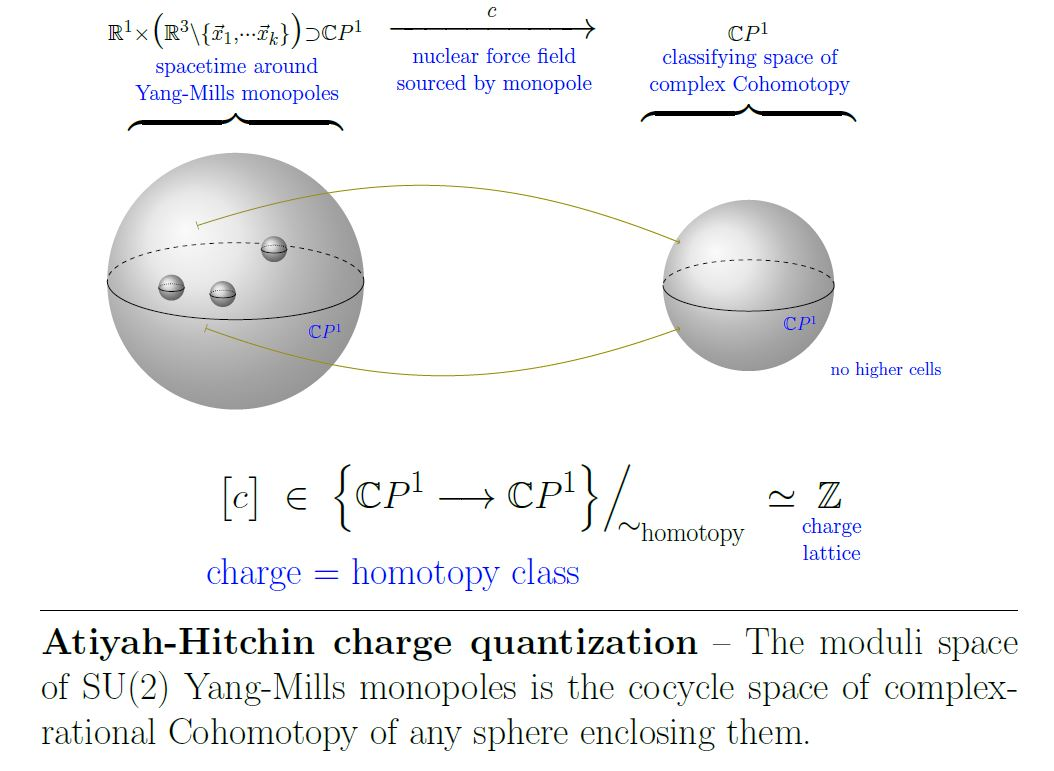This is a non-abelian analog of the Dirac charge quantization of the electromagnetic field, with ordinary cohomology replaced by Cohomotopy cohomology theory.

## Examples

### Cohomotopy in the lowest dimensions

Since the 0-sphere is the disjoint union of two points, $0$-cohomotopy corresponds to the powerset of the connected components of a space.

Further, the $(-1)$-sphere is understood as the empty space. Since the only map to the empty space is the identity map from the empty space, the $(-1)$-cohomotopy of a space is measuring whether that space is empty. In the context of homotopy type theory, this is the same as negation.

### Of projective spaces

Cohomotopy sets of projective spaces are computed in West 71

### Of 4-Manifolds

Let $X$ be a 4-manifold which is connected and oriented.

The Pontryagin-Thom construction as above gives for $n \in \mathbb{Z}$ the commuting diagram of sets

$\array{ \pi^n(X) &\overset{\simeq}{\longrightarrow}& \mathbb{F}_{4-n}(X) \\ {}^{ \mathllap{h^n} } \downarrow && \downarrow^{ h_{4-n} } \\ H^n(X,\mathbb{Z}) &\underset{\simeq}{\longrightarrow}& H_{4-n}(X,\mathbb{Z}) \,, }$

where $\pi^\bullet$ denotes cohomotopy sets, $H^\bullet$ denotes ordinary cohomology, $H_\bullet$ denotes ordinary homology and $\mathbb{F}_\bullet$ is normally framed cobordism classes of normally framed submanifolds. Finally $h^n$ is the operation of pullback of the generating integral cohomology class on $S^n$ (by the nature of Eilenberg-MacLane spaces):

$h^n(\alpha) \;\colon\; X \overset{\alpha}{\longrightarrow} S^n \overset{generator}{\longrightarrow} B^n \mathbb{Z} \,.$

Now

• $h^0$, $h^1$, $h^4$ are isomorphisms

• $h^3$ is an isomorphism if $X$ is “odd” in that it contains at least one closed oriented surface of odd self-intersection, otherwise $h^3$ becomes an isomorphism on a $\mathbb{Z}/2$-quotient group of $\pi^3(X)$ (which is a group via the group-structure of the 3-sphere (SU(2)))

flavours of
Cohomotopy
cohomology theory
cohomology
(full or rational)
equivariant cohomology
(full or rational)
non-abelian cohomologyCohomotopy
(full or rational)
equivariant Cohomotopy
twisted cohomology
(full or rational)
twisted Cohomotopytwisted equivariant Cohomotopy
stable cohomology
(full or rational)
stable Cohomotopyequivariant stable Cohomotopy

(equivariant) cohomologyrepresenting
spectrum
equivariant cohomology
of the point $\ast$
cohomology
of classifying space $B G$
(equivariant)
ordinary cohomology
HZBorel equivariance
$H^\bullet_G(\ast) \simeq H^\bullet(B G, \mathbb{Z})$
(equivariant)
complex K-theory
KUrepresentation ring
$KU_G(\ast) \simeq R_{\mathbb{C}}(G)$
Atiyah-Segal completion theorem
$R(G) \simeq KU_G(\ast) \overset{ \text{compl.} }{\longrightarrow} \widehat {KU_G(\ast)} \simeq KU(B G)$
(equivariant)
complex cobordism cohomology
MU$MU_G(\ast)$completion theorem for complex cobordism cohomology
$MU_G(\ast) \overset{ \text{compl.} }{\longrightarrow} \widehat {MU_G(\ast)} \simeq MU(B G)$
(equivariant)
algebraic K-theory
$K \mathbb{F}_p$representation ring
$(K \mathbb{F}_p)_G(\ast) \simeq R_p(G)$
Rector completion theorem
$R_{\mathbb{F}_p}(G) \simeq K (\mathbb{F}_p)_G(\ast) \overset{ \text{compl.} }{\longrightarrow} \widehat {(K \mathbb{F}_p)_G(\ast)} \!\! \overset{\text{Rector 73}}{\simeq} \!\!\!\!\!\! K \mathbb{F}_p(B G)$
(equivariant)
stable cohomotopy
$K \mathbb{F}_1 \overset{\text{Segal 74}}{\simeq}$ SBurnside ring
$\mathbb{S}_G(\ast) \simeq A(G)$
Segal-Carlsson completion theorem
$A(G) \overset{\text{Segal 71}}{\simeq} \mathbb{S}_G(\ast) \overset{ \text{compl.} }{\longrightarrow} \widehat {\mathbb{S}_G(\ast)} \!\! \overset{\text{Carlsson 84}}{\simeq} \!\!\!\!\!\! \mathbb{S}(B G)$

### General

Original articles:

Further discussion of Cohomotopy:

### Pontrjagin-Thom construction

#### Pontrjagin’s construction

##### General

The Pontryagin theorem, i.e. the unstable and framed version of the Pontrjagin-Thom construction, identifying cobordism classes of normally framed submanifolds with their Cohomotopy charge in unstable Borsuk-Spanier Cohomotopy sets, is due to:

(both available in English translation in Gamkrelidze 86),

as presented more comprehensively in:

The Pontrjagin theorem must have been known to Pontrjagin at least by 1936, when he announced the computation of the second stem of homotopy groups of spheres:

• Lev Pontrjagin, Sur les transformations des sphères en sphères (pdf) in: Comptes Rendus du Congrès International des Mathématiques – Oslo 1936 (pdf)

Review:

Discussion of the early history:

##### Twisted/equivariant generalizations

The (fairly straightforward) generalization of the Pontrjagin theorem to the twisted Pontrjagin theorem, identifying twisted Cohomotopy with cobordism classes of normally twisted-framed submanifolds, is made explicit in:

A general equivariant Pontrjagin theorem – relating equivariant Cohomotopy to normal equivariant framed submanifolds – remains elusive, but on free G-manifolds it is again straightforward (and reduces to the twisted Pontrjagin theorem on the quotient space), made explicit in:

• James Cruickshank, Thm. 5.0.6, Cor. 6.0.13 in: Twisted Cobordism and its Relationship to Equivariant Homotopy Theory, 1999 (pdf, pdf)
##### In negative codimension

In negative codimension, the Cohomotopy charge map from the Pontrjagin theorem gives the May-Segal theorem, now identifying Cohomotopy cocycle spaces with configuration spaces of points:

• Peter May, The geometry of iterated loop spaces, Springer 1972 (pdf)

• Graeme Segal, Configuration-spaces and iterated loop-spaces, Invent. Math. 21 (1973), 213–221. MR 0331377 (pdf)

c Generalization of these constructions and results is due to

• Dusa McDuff, Configuration spaces of positive and negative particles, Topology Volume 14, Issue 1, March 1975, Pages 91-107 (doi:10.1016/0040-9383(75)90038-5)

• Carl-Friedrich Bödigheimer, Stable splittings of mapping spaces, Algebraic topology. Springer 1987. 174-187 (pdf, pdf)

#### Thom’s construction

Thom's theorem i.e. the unstable and oriented version of the Pontrjagin-Thom construction, identifying cobordism classes of normally oriented submanifolds with homotopy classes of maps to the universal special orthogonal Thom space $M SO(n)$, is due to:

Textbook accounts:

#### Lashof’s construction

The joint generalization of Pontryagin 38a, 55 (framing structure) and Thom 54 (orientation structure) to any family of tangential structures (“(B,f)-structure”) is first made explicit in

and the general statement that has come to be known as Pontryagin-Thom isomorphism (identifying the stable cobordism classes of normally (B,f)-structure submanifolds with homotopy classes of maps to the Thom spectrum Mf) is Lashof 63, Theorem C.

Textbook accounts:

Lecture notes:

• John Francis, Topology of manifolds course notes (2010) (web), Lecture 3: Thom’s theorem (pdf), Lecture 4 Transversality (notes by I. Bobkova) (pdf)

• Cary Malkiewich, Section 3 of: Unoriented cobordism and $M O$, 2011 (pdf)

• Tom Weston, Part I of An introduction to cobordism theory (pdf)

### Examples

Cohomotopy sets of projective spaces:

• Robert West, Some Cohomotopy of Projective Space, Indiana University Mathematics Journal Vol. 20, No. 9 (March, 1971), pp. 807-827 (jstor:24890146)

Cohomotopy sets of 4-manifolds:

Cohomotopy sets of Thom spaces:

In relation to quaternionic line bundles over 5-manifolds:

Cohomotopy sets of spin-manifolds in co-degree 1:

### Cohomotopy cocycle spaces

Discussion of Cohomotopy cocycle spaces (i.e. spaces of maps into an n-sphere):

• Vagn Lundsgaard Hansen, The homotopy problem for the components in the space of maps on the $n$-sphere, Quart. J. Math. Oxford Ser. (3) 25 (1974), 313-321 (DOI:10.1093/qmath/25.1.313)

• Vagn Lundsgaard Hansen, On Spaces of Maps of $n$-Manifolds Into the $n$-Sphere, Transactions of the American Mathematical Society, Vol. 265, No. 1 (May, 1981), pp. 273-281 (jstor:1998494)

• Victor Vassiliev, Twisted homology of configuration spaces, homology of spaces of equivariant maps, and stable homology of spaces of non-resultant systems of real homogeneous polynomials (arXiv:1809.05632)

• Douglas Ravenel, What we still don’t know about loop spaces of spheres, in: Mark Mahowald, Stewart Priddy (eds.), Homotopy Theory via Algebraic Geometry and Group Representations, Contemporary Mathematics 220, AMS 1998 (pdf, pdf, doi:10.1090/conm/220)

Discussion of cocycle spaces for rational Cohomotopy (see also at rational model of mapping spaces):

• Jesper Møller, Martin Raussen, Rational Homotopy of Spaces of Maps Into Spheres and Complex Projective Spaces, Transactions of the American Mathematical Society Vol. 292, No. 2 (Dec., 1985), pp. 721-732 (jstor:2000242)

### In computational topology

Discussion of Cohomotopy-sets in computational topology:

### Cohomotopy in topological data analysis

The suggestion to regard cobordism theory of iso-hypersurfaces, and thus Pontryagin's theorem in Cohomotopy, as a tool in (persistent) topological data analysis (improving on homologuical well groups):

### Equivariant Cohomotopy

Discussion of the stable cohomotopy (framed cobordism cohomology theory) in the equivariant cohomology-version of cohomotopy (equivariant cohomotopy):

and in the twisted cohomology-version (twisted cohomotopy)

Discussion of M-brane physics in terms of rational equivariant cohomotopy:

and in terms of twisted cohomotopy:

### In spectral geometry

Discussion of smooth functions into the 4-sphere in the context of Connes-Lott models in spectral non-commutative geometry: